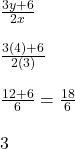## What is the value of the expression: 3y + 6 divided by 2x, if y = 4 and x = 3? *

Question

What is the value of the expression: 3y + 6 divided by 2x, if y = 4 and x = 3? *

in progress 0
7 months 2021-07-28T04:42:22+00:00 1 Answers 9 views 0